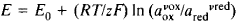# Electrode Potential

Also found in: Dictionary, Wikipedia.
Related to Electrode Potential: Standard electrode potential

## electrode potential

[i′lek‚trōd pə′ten·chəl]
Also known as electrode voltage.
(electronics)
The instantaneous voltage of an electrode with respect to the cathode of an electron tube.
(physical chemistry)
The voltage existing between an electrode and the solution or electrolyte in which it is immersed; usually, electrode potentials are referred to a standard electrode, such as the hydrogen electrode.
McGraw-Hill Dictionary of Scientific & Technical Terms, 6E, Copyright © 2003 by The McGraw-Hill Companies, Inc.
The following article is from The Great Soviet Encyclopedia (1979). It might be outdated or ideologically biased.

## Electrode Potential

the difference in electric potential between an electrode and the electrolyte with which it is in contact (usually, between a metal and an electrolyte solution).

An electrode potential is due to the transfer of charged particles across a phase boundary, to the specific adsorption of ions, or, when polar molecules are present (including those of a solvent), to the polar adsorption of the molecules. The magnitude of the electrode potential in a nonequilibrium state depends both on the nature and composition of the phases in contact and on the kinetic behavior of the electrode reactions at the phase boundary. The equilibrium value of the jump in potential at an electrode-solution boundary is determined exclusively by the specifics of the electrode reaction and is independent of the nature of the electrode and its adsorption of surfactants. This absolute potential difference between points located in two different phases cannot be measured experimentally or calculated theoretically. Relative electrode potentials have practical significance. Usually called simply electrode potentials, they represent the difference between the electrode potential of the electrode in question and that of a reference electrode—most often a normal hydrogen electrode, whose electrode potential is assumed by convention to be equal to zero.

When there is electrochemical equilibrium at the electrode, the value of the electrode potential E can be expressed in terms of the change in the Gibbs free energy ΔG of the reaction: E = –ΔG/zF, where z is the number of electrons involved in the electrochemical process and F is the Faraday constant. In this case the electrode potential depends on the activity a of the substances involved in the reaction (potential-determining substances). For Me/Men+ electrodes E = E0 + (RT/zF) In aMen+, where R is the gas constant, T is the temperature, and E0 is the standard potential. For oxidation-reduction with inert electrodes, in which all components of the electrochemical reaction are in solution, the electrode potential (oxidation-reduction potential) is determined by the activity both of the oxidized form aox and the reduced form ared of the substance:where v is the stoichiometric coefficient.

If the simultaneous occurrence of more than one electrode reaction is possible at an electrode, the concept of a steady-state potential is used. When an electric current is passed, the measured electrode potential will differ from the equilibrium potential by the amount of polarization (seePOLARIZATION, ELECTROCHEMICAL).

V. V. GORODETSKII

The Great Soviet Encyclopedia, 3rd Edition (1970-1979). © 2010 The Gale Group, Inc. All rights reserved.
References in periodicals archive ?
Equation (1) can now be expressed in terms of electrode potential by substituting for IG and IG0
Similarly for the end plate, the net anodic current density per unit length of corrosion couple [i.sub.a](x) on end plate at an electrode potential [E.sub.x] is
(1) In acid solution, the electrode potential increases with rising pH, in the same way that is known of the hydrogen oxidation at a hydrogen electrode.
This value was determined by varying the current density until reaching the electrode potential determined by the microelectrolysis experiments, which was within -1.15 [less than or equal to] E [less than or equal to] -0.95 V/SHE.
In this case, the electrode potential did not drop to the lithium intercalation potential while the graphite electrode was charged to 20 mAh x [g.sup.-1] in the same manner as shown in Figure 3(a).
Further analysis of base voltammograms obtained by a continuous increase of the forward potential scan limit expressed through the dependency of the charge associated with anodic film formation from the electrode potential is presented in Figure 2.
The design of the proposed moisture meter based on SICR is such that it does not contain a structural dielectric material supporting the inner electrode potential. Structurally, the inner electrode potential tightly connected to the outer electrode and the test liquid is in the space between the electrodes.
At the point where the maximum power has occurred ohmic resistance and electrode potential have reached their maximum size and due to this, after this stage, power density is reduced.
End point was determined by a sudden rapid change in electrode potential.
The pH of the electrolyte influences reaction kinetics at the individual electrodes, as well as the electrode potential at which oxidation or reduction occurs [20-24].
The electrode potential of the reference electrode did not change in the process of measuring.

Site: Follow: Share:
Open / Close